﻿ Sensible Calculus V.A

# V. The Definite Integral (draft)

Dec., 2005: This page now requires Internet Explorer 6+MathPlayer or Mozilla/Firefox/Netscape 7+.
Preface: The definition of the derivative articulates a concept used in understanding rates of change in several different contexts where one variable "controls" the value of another variable. In chapter IV our examination of differential equations demonstrated that knowing the derivative (how fast we are traveling on a highway) can help us determine its primitive function (our location or change in position as a function of time). In another context, knowing how fast the rain is falling allows us to find the watershed volume or water level (change in water volumes or heights) as functions of time. The problem of solving differential equations was investigated using graphical tools (tangent fields and integral curves), numerical tools (Euler's method for estimating the values of solutions with initial conditions- a numerical integration) and, for a more limited family of differential equations, symbolically (indefinite integrals).

In finding exactly and estimating functions to solve differential equations we have seen another concept taking shape to understand change. In somewhat abstract terms, we want to find the net change in the value of a controlled (dependent) variable for a given interval of the controlling (independent) variable. The consolidation (integration) of information about a controlled variable's rate of change,  `{dy}/{dx}=f '(x)`, with respect to a single controlling variable, x, for the interval [a,b],  leads to the recovery of information about the net change in the controlled variable's values, `Delta y = f (b) – f(a)`. This context is common enough to articulate it more carefully and distinguish it from those already developed  in solving differential equations.

In our study we found that by accumulating estimates of change in a controlled variable (dy ` ~~ Delta`y) over small intervals of change in the controlling variable (dx = `Delta`x) we could make reasonable estimates of the net change in the controlled variable over a longer interval ( [a,b]). In this chapter we will define and begin to study the concept called the definite integral that captures this procedure of accumulating estimates and the number estimated by this process.

V. A. A[n Informal] Definition of The Definite Integral (Euler)
 The sums used in Euler's method (Chapter IV.***) to estimate the solution to a differential equation of the form f '(x) = P(x) where P was a continuous function had at least two interpretations, one related to motion and one related to areas.  In the motion interpretation these sums estimated the net change in a moving object's position, S, during the time interval [a,b], i.e., S(b) - S(a), based on the model assumption that its velocity at time t was given by  v(t) = S'(t) = P(t). See Figure 1.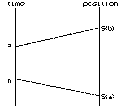Figure 1 In the geometric interpretation when P(x) > 0  the sums estimated the area of the region in the coordinate plane enclosed by the X axis, the lines X = a and X = b, and the graph of the function P, i.e., the graph of the equation Y = P(x). See Figure 2. The fact that these sums appear to approach a single number as their limit and the importance of these sums in a variety of interpretations has led to the articulation of the mathematical concepts involved.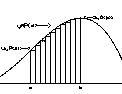Figure 2
Overview: The abstraction that has developed from these and other interpretation of these estimations focuses on the limiting number, called the definite integral of the function P over the interval [a,b]. As we proceed to give a[n informal] definition of this concept, keep in mind that we are describing the specific number which is the limit of these estimating sums. As the number of summands increases the estimating sums approach a single number which can be interpreted in the two distinct models using the function P to describe either the velocity of a moving object or the graph of a function bounding of a planar region. The definition with which we begin works perfectly well for continuous functions on compact (closed and bounded) intervals, so we will use it initially to describe the key properties of the definite integral and its applications. Later we will explore how the definition we have given can be extended to allow more general considerations on estimating this number and also to overcome some more subtle problems with functions that are not continuous (step functions and functions with vertical asymptotes) or for intervals that are not compact (bounded, open intervals and unbounded intervals).

Using our work in Chapter IV as a guide, we first establish some notation.

Notation: Suppose that P is a function defined on the interval [a,b] and N is a positive integer. We will use let dx  = `Delta x =h =(b-a)/N`.  With this established  we continue by denoting a by x 0, so a = x 0, and then we let x 1 = a + `Delta`x,

x 2= x 1 + `Delta`x = a + 2 `Delta`x ,

x 3= x 2 + `Delta`x = a + 3 `Delta`x .

We continue this notation simply by letting x k = a + k `Delta`x. Note that this means that x N = a + N `Delta`x = b.
Since the numbers x k arose in the context of Euler's method for solving a differential equation,
we describe the set {`a = x_0 , x_1 , x_2 , ... , x_N =b`} as an Eulerian partition of the interval [a,b] with norm or mesh dx  = `Delta x =h =(b-a)/N`.

Notes:

1. We have assumed that a<b, so that dx = `Delta`x = h > 0 and thus `a = x_0 < x_1 < x_2 < ... < x_N =b`.

2. As the size of N increases, the size of `Delta`x = h approaches 0. Symbolically, as N ` -> oo, Delta x = h -> 0`.

3. If we had chosen a and b without the presumption that a<b, all the notation would still make sense, though if b<a then `Delta`x<0 and
`b = x_N < x_{N-1} <  ... < x_1 < x_0 =a`.

As we have said before, sums were important quantities in estimating the net change in position and the area in our previous work, so we establish a shorthand notation for these important numbers as well by letting

`S(P,N) -=  P(x_0) Delta x + P(x_1) Delta x + ... + P(x_{N-1}) Delta x `   (*)

We'll refer to these sums as the Nth Eulerian sum for the function P over the interval [a,b] or the Eulerian sum for short.

 More Notes: 1. The Eulerian partition of [a,b] breaks the interval into N distinct segments (sub- intervals). In the Eulerian sums we evaluate the function P at the left hand endpoint of each of these intervals. The left hand endpoint of the first segment is x 0, the left hand endpoint of the second segment is x 1, the left hand endpoint of the third segment is x 2, and the pattern continues with the left hand endpoint of the kth segment being xk - 1, so that last summand of S(P,n) evaluates P at the left-hand endpoint of the (last) Nth segment, namely xN - 1.  2. The Democracy Principle : "What's good for one is good for all."  Essentially the sum is the accumulation of the results of the same computational process applied to each subinterval: Evaluate P at the left hand endpoint of the segment, then multiply that result by `Delta`x. This is one of the key principles for all of mathematics! Historical Comment: The precise definition of the definite integral has had a long history and as with many mathematical concepts, with its continued refinement it has gained in abstraction and power. It was Augustin Cauchy who is generally credited with bringing some precision to these concepts in the early 19th century, but the development continued to its generally acknowledged first completion in the mid 19th century by the German mathematician Georg Friedreich Bernhard Riemann (1826 -1866). Only in the beginning of the 20th century did the definite integral reach its full generality with the work of the French mathematician Henri Lebesgue (1875-1941) on what is now described as the theory of measure. The notion of the definite integral has continued to grow further even in the 20th century, demonstrating the vitality of the concept in an ever expanding world of mathematical studies.
3. As we have seen in Chapter IV, it is sometimes simpler for computational purposes to use the formula
`S(P,N)  =  [ P(x_0) + P(x_1) + ... + P(x_{N-1})] Delta x `.

which results from recognizing the common factor of `Delta`x in equation *.

4. Although the notation ignores the relation between the number N and the other subscripts used in denoting the numbers in the partition of [a,b], don't be fooled into thinking that the partitioning numbers are the same for different N. For example, for the interval [0,5] the Euler partition with N = 5  is {0,1,2,3,4,5} so with N= 5 it turns out that xk = k. But with N=4, the Euler partition is {`0, 5/4, 5/2, 15/4, 5`} or xk = `(5k)/4`.
The notation ignores the different values of N, but it is important to recognize this, since it means that data used to compute with N= 5 will be different from that used to compute with N=4.
If we wish to use data computed when N=5 in the computation of S(P,N) for other values of N, 5 must be a factor of N.
Here is another place where bisection or decimation can come in handy as a technique for computing that does not discard old information.

 5. Our treatment here has emphasized the motion and area interpretations of the definite integral, but we should not forget that the differential equation tangent field also can be used to visualize these sums. In particular we can interpret S(P,N) using a tangent field for the differential equation `(dy)/(dx) = P(x)` and estimating an integral curve for this field with line segments. See Figure 3.  We can consider this estimate as an approximation for the graph of a position function s that satisfies the differential equation. Now locate the points on the estimating curve with first coordinates a and b. Assume these points have coordinates (a,c) and (b,d). The interpretation of the sum as the net change in position is now seen as the accumulation of the step by step vertical changes made by this estimating curve over the interval [a,b].  Thus we see as well that `S(P,N) ~~ d - c` and represents an estimate of the net change in the second coordinates for an integral curve fitting this field.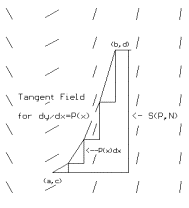Figure 3
6. The Party Principle: "The more the merrier." By taking N larger, the number of summands increases.
Our experience with the interpretations of motion and area suggest that the sums will approach a specific number.
This suggests that the more subintervals and data computed, the closer the sum will come to the limiting value.

Example V.A.1. In this example we consider some specifics in computing the Euler sums.
Suppose P(x) = 3 x 2  and a = 1 while b = 5 with N = 4. Then `Delta x = (5 -1)/4 = 1` and so x 0 = 1 , x 1 = 2, x 2 = 3 , x 3 = 4, and x 4 = 5.

Thus, S(P,4) = P(x 0) `Delta`x + P(x 1) `Delta`x + P(x 2)`Delta`x + P(x 3)`Delta`x

=   3    +    12     +     27   +      48    = 90.

Using  N = 5, gives `Delta x = (5 -1)/5 = 4/5 `, so x 0 = 1 , x 1 = `9/5`, x 2 = `(13)/5` , x 3 = `(17)/5`, x 4 = `(21)/5`, and x 5 = 5.

Finally, S(P,5) = [P(x 0) + P(x 1) + P(x 2) + P(x 3) + P(x 4)] `Delta x = [3 + 3 (81)/25 + 3 (169)/25  + 3((17)/5)^2  +3((21)/5)^2 +75](4/5) ~~ 98.88`.

 x 3x2 1 3 2 12 3 27 4 48 euler  sum = 90

 x 3x2 1 3 1.8 9.72 2.6 20.28 3.4 34.68 4.2 52.92 euler sum = 98.88

Using N = 40, gives `Delta x = (5 -1)/40 =1/10`.  This N decimates the interval with segments of length 0.1 so the partition is {1, 1.1, 1.2, 1.3, 1.4, ... ,4.8, 4.9, 5} Finding the values of P at 40 numbers is something best left to some kind of calculating program or spread sheet. It can also be computed using some clever algebra , but however it is done, the sum `S(P,40)~~120.42`.

Of course much larger values of n would be even more difficult without the aid of a calculating procedure or better algebra.
Here is a table showing the results of further computation of S(P,N) in a decimating scheme.

 N `Delta`x S(P,N) 40 0.1 120.42 400 0.01 123.6402 4000 0.001 123.964002 40000 0.0001 123.99640002 400000 0.00001 123.99964 4000000 0.000001 123.999964
It seems clear from this table that as N gets larger, the values of S(P,N) get close to 124.
Recall that in Chapter IV we saw in the Fundamental Theorem of Calculus that
these sums would be approaching F(5)-F(1) for any function F where F '(x) = P(x) = 3x2.
Using F(x) = x3 we see that the table is not misleading in its values since 53 -1 = 124

(Informal) Definition: The Definite Integral - Euler: Suppose P is a function defined on [a,b] and S(P,N) is defined as above. As we have seen in  Chapter IV, it is often the case that when N is very large the values of S(P,N) approach the number I. In this case we say that P is (Euler) integrable over the interval [a,b] and we call I the definite integral of P over the interval [a,b] (or I  is the definite integral of P from a to b).  In this situation we denote I by the following symbols ( due to Liebniz):
I = `int_a^b  P  dx  =  int_a^b  P(x)dx`

The work of chapter IV  suggests two results that we record here with a brief review of the evidence we have for their being true.
Theorem V.1. (Continuity implies Integrability) If P is a continuous function on [a,b] then P is integrable.

Theorem V.2 (The Fundamental Theorem of Calculus - Evaluation Form)

Suppose P is a continuous function on [a,b] and
F is a differentiable function on [a,b] with F '(x) = P(x) for all x in (a,b). Then

I = ` int_a^b  P(x)dx` = F(b)F(a)

Evidence for Theorems V.1 and V.2:

 Motion Interpretation. Consider P as a velocity function which changes continuously for the time interval [a,b].  We can consider the sums S(P,N) as the result of selecting the velocity values at a finite number of points in the time interval, using these velocities to estimate the change in position for short time intervals, and then accumulating these estimates for an estimate of the net change in position for an object moving with velocity P for the time interval [a,b]. Since the velocity is a continuous function we can perform a thought experiment setting controls for an object to move with the given velocities. When the object in this experiment moves we can keep track of its position with the function F. Its  net change in position  for the time interval [a,b] will be the number F(b)-F(a). This is the number  that the sums S(P,N)  approach when N is very large. Cost Interpretation. Consider P as a marginal cost function which changes continuously for the production level interval [a,b].  We can consider the sums S(P,N) as the result of selecting the marginal cost values at a finite number of points in the proction level interval, using these marginal costs to estimate the change in cost for short production level intervals, and then accumulating these estimates for an estimate of the net change in costs for production for increasing the production over the interval [a,b]. Since the marginal cost is a continuous function we can perform a thought experiment setting controls for production to change with the given marginal costs. When production in this experiment changes we can keep track of its total costs with the function F. Its  net change in costs  for the production interval [a,b] will be the number F(b)-F(a). This is the number  that the sums S(P,N)  approach when N is very large.
Area Interpretation. When P(x) > 0 for all x between a and b, then the summands of S(P,N) can be thought of as the areas of rectangles  that are accumulated to give an estimate of the area of the region in the plane bounded by the graph of the function P, the lines X=a, X=b and  the X-axis. Since this region seems well contained by its continuous boundary, it seems reasonable that we can measure its area, and thus the sums are approaching this measurement.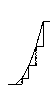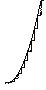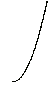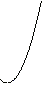This  supports Theorem V.1's result on the existence of a limiting number for the Euler sums.
Integral Curve Interpretation:
We form polygonal curves shaped by lines segments drawn to match the tangent field for the differential equation `(ds)/(dt) = P(t)`. In particular we can interpret `int_a^b P(x) dx`  using this tangent field and choosing any integral curve for this field. We can consider this curve as a graph of a position function s that satisfies the differential equation. See Figure 5. Now locate the points on the curve with first coordinates a and b. These points have coordinates (a,s(a)) and (b,s(b)).

The sums accumulate estimates of changes in the second coordinates of the endpoint of these curves over the interval [a,b]. Since P(t) is continuous we can conceive of directing a drawing instrument to match the differential equation's tangent requirements at every point of its drawing. This results in drawing polygonal curves shaped by line segments drawn to match the differential equation `(ds)/(dt) = P(t)` with an integral curve which the polygonal curves approximate. See Figures 4 and 5.  The interpretation of the definite integral as the net change in position is now seen as the change s(b)-s(a). That is, the definite integral represents the vertical change in the integral curve over the interval [a,b]. The net change in the second coordinates of this integral curve is the number which the sums are approaching.Figure 4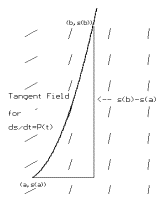Figure 5

Example V.A.1. (Cont'd.) With this new notation we can say that the value of  S(P,40) = 120.42 is an estimate for `int_a^b P(x)dx = int_1^5 3x^2 dx`.
Using the Fundamental Theorem of Calculus with F(x) = x 3  we can express our previous conclusion about the value of this definite integral with the equation
`int_1^5 P(x)dx = int_1^5 3x^2 dx = F(5) - F(1) = 5^3 -1^3 = 124 `

A Little More Notation: As we have seen in chapter IV, the evaluation of the difference  F(b) - F(a) is both useful and common. For this reason the older notation for evaluation of a variable has been extended to denote this difference by `F(x)| _{x=a}^{x=b} =  F(x) | _a^b  =  F(b)  -  F(a)`. So for example in the last example the work might have omitted the naming of the function F by displaying F(x) instead as follows:

`int_1^5 P(x)dx = int_1^5 3x^2 dx = x^3| _1^5 = 5^3 -1^3 = 124 `

1. Can we always find an anti-derivative for P as an elementary function?

This would be quite nice, matching the niceness of the derivative calculus where we have rules that allow us to find the derivative of any elementary function. Unfortunately this is not the case. Functions as simple as `sin(x^2)` and ` e^{-x^2}` do not have antiderivatives that can be expressed as an elementary function. [The proof of this fact is not easy.] As a result there is no easy way to find `int_0^1 sin(x^2) dx`  or `int_0^1 e^{-x^2} dx`  by applying Theorem V.2.

2. Is there a geometric interpretation for the definite integral when the integrand Q is negative on the interval [a,b]?

 Yes. If we consider a function Q that is negative on the interval [a,b], it's opposite, P = -Q, will be always  positive. See Figure 6.  The graph of its opposite, P, will enclose a region above the X-axis that has the same area as the region enclosed by the graph of Q, the X-axis, and the lines X = a and X = b. But the sums in the estimates for `int_a^b Q(x) dx`  will be opposite the estimates for `int_a^b P(x) dx`. So we can say that the definite integral for Q will be the opposite of the integral for P. Since the integral for P  measures the area of the region, the integral for Q must be the opposite of the area of the region enclosed by the graph of Q, the X-axis, and the lines X = a and X = b.  So ... For intervals [a,b] where an integrand Q is negative,`-int_a^b Q(x) dx`  is the area of the region enclosed by the graph of Q, the X-axis, and the lines X = a and X = b.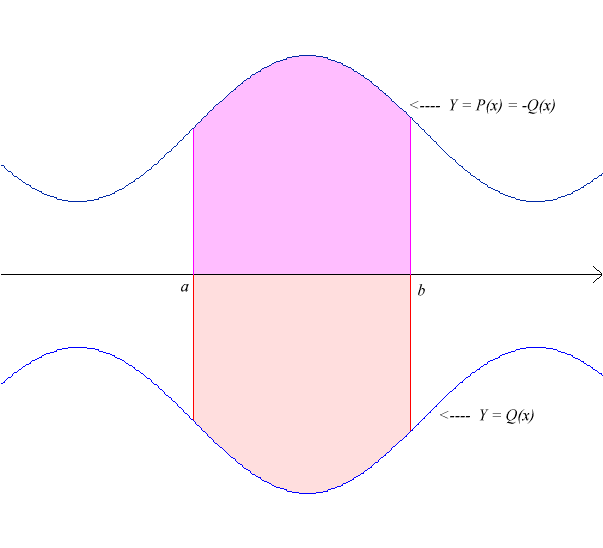Figure 6

Exercises V.A.

1. Find  each of the following definite integrals using the Fundamental Theorem of Calculus.
1.   `int_0^1 x^2 + 4x^5 dx`
2.   `int_1^2 3x + 5/(x^2) dx`
3.   `int_1^2 1/(x^3) dx`
4.   `int_1^8 x^{1/3} dx`
5.   `int_4^9 -x/2 + sqrt(x) dx`
2. Estimate the following definite integrals with Euler sums a) with n = 4 , b) with n = 8 and c) n = 100 (using technology). Compare your estimates with the exact values using problem 1. Explain briefly the quality of your estimate.
1.   `int_0^1 x^2 + 4x^5 dx`
2.   `int_1^2 3x + 5/(x^2) dx`
3.   `int_1^2 1/(x^3) dx`
4.   `int_1^8 x^{1/3} dx`
5.   `int_4^9 -x/2 + sqrt(x) dx`
3. Estimate the following definite integrals with Euler sums a) with n = 4 , b) with n = 8 and c) n = 100 (using technology). When possible compare these estimates with the value obtained for the integral by using the fundamental theorem of calculus.
1.   `int_0^1 1 + x dx`
2.   `int_0^1 1 + x^2 dx`
3.   `int_0^1 x^3 dx`
4.   `int_0^1 1/(1+x) dx`
5.   `int_1^2 1/x dx`
6.   `int_0^1 1/(1 + x^2) dx`
4. For each function P on the interval [1,5], give an interpretation for the sum S(P,4)  a) as an area of a region in the plane b) as the net change in position of a moving object, and c) as the net change in the second coordinates of two points and a polygonal curve estimating a solution for a differential equation. Draw any suitable graphs and figures to illustrate your interpretations.
1. `P(x) = 2x -1`
2. `P(x) = 1/x`
3. `P(x) = x^2`
5. Give an interpretation with a related visualization for the following integrals a) as an area of a region in the plane b) as the net change in position of a moving object, and c) as the net change in the second coordinates of two points and an integral curve for a differential equation.
1.   `int_0^1 1/(1 + x) dx`
2.   `int_0^1 1/(1 + x^2) dx`
3.   `int_1^2 1/x dx`
6. Suppose f is a continuous probability density function for a random variable X on the interval [a,b].  The probability that X will lie between the numbers A and B with A<B can be estimated by the product f(A) (B-A).
1. Discuss the ability to estimate the probability that X will lie between A and B by the euler sum, S(f , N) for [A,B].
2. Based on part A) explain why the probability that X lies between A and B is `int_a^b f(x) dx`.
3. Suppose an object is moving with its velocity given by v(t) for time t between a and b. Discuss the relation between estimating the object's position at time b from its position at time a using Euler's Method and is equal to `int_a^b v(t) dt`.
7. Use the fact that `d/(dt) ln(t) = 1/t` for t >0 to explain why `ln(2) = int_1^2 1/t dt`. Estimate ln(2) using euler sums for the interval with

8. N= 4, N= 10, and N= 100. Draw a figure that illustrates this estimation as an area problem. Discuss the relation of ln(x) for x>1 to a definite integral .

Appendix: Other ways to define the Definite Integral for a continuous function.

When considering continuous functions over a compact interval, several alternative definitions can be used to estimate the same number that we have defined using the left endpoint Euler sums.
Here a some of those alternatives with figures (to be added still!) illustrating their meaning in terms of both the motion and the area interpretations of the estimates.

 Euler: Let `Delta x = (b - a)/n`  ;   `x_ k = a + k Delta x`, `k = 0, 1,... n.`          and  `S _n(P,n) -= sum _{k=0}^{k = n-1}  P(x _k) Delta x`. Then we define the definite integral by `int_a^b P(x)d x  -=  lim _{n -> oo}  S_n (P,n)`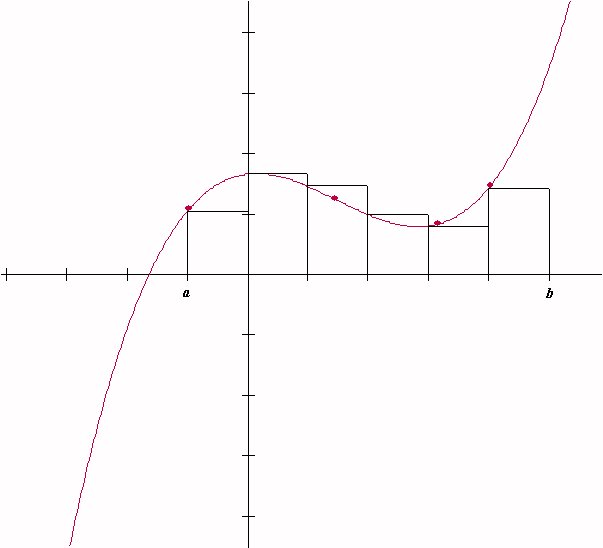Extended Euler:  Choose numbers `w_k`  so that  `x_{k-1}<= w_k <= x_k ;  k = 1, ..., n` . Let W = { w 1, w 2, ... ,w n-1, w n }. Now we let  `S _n (P,n,W) -= sum _{k=1}^{k = n}  P(w _k) Delta x`. Then we define the definite integral by   `int_a^b P(x)d x  -=  lim _{n -> oo}  S_n (P,n,W)`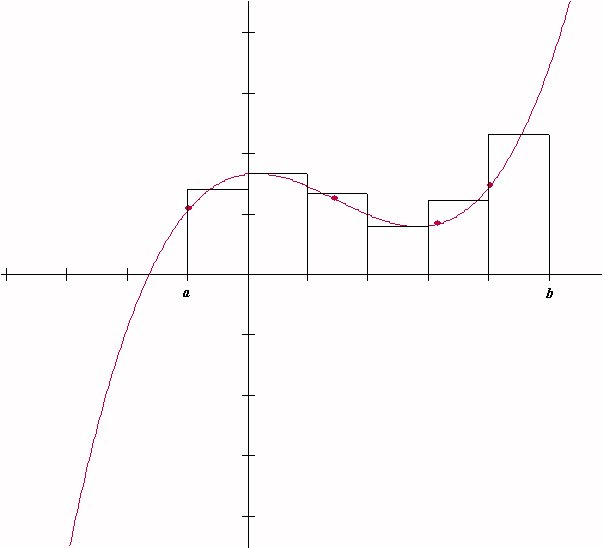Riemann: Start with a partition V of numbers from the interval [a,b], `V = { a = x_0 <=x_1<=x_2<=...<=x_{n-1}<=x_n=b}`.
Let
`Delta`x k = x k - x k-1Now choose numbers wk  so that  `x_{k-1}<= w_k <= x_k ;  k = 1, ..., n` .

Let W = { w 1, w 2, ... ,w n-1, w n }and let `Delta`x = mesh of the partition V = max {`Delta`x k , k = 1 to n}
Now we let   `S _n (P,W) -= sum _{k=1}^{k = n}  P(w _k) Delta x_k`. Then we define the definite integral by

`int_a^b P(x)d x  -=  lim _{Delta x -> 0}  S_n (P,W)`

Without Limits: Let `M_k = `sup `{ P(x), x_{k-1} <= x <= x_k }`  [maximum when f is continuous] and
` m_k =` inf `{ P(x), x_{k-1} <= x <= x_k }`  [minimum when f is continuous].
Using these we continue to define `U_n (P,n) = sum _{k=1}^{k=n} M_k  Delta x_k` [The Upper Riemann Sum] and
`L_n (P,n) = sum _{k=1}^{k=n}  m_k Delta x_k` [The Lower Riemann Sum]. Then we define the definite integral by

`int_a^b P(x) dx -= ` I,  a unique number with the property that for any partition,  `L_n (P,n)  <=`  I ` <= U_n (P,n)`.

Using an Antiderivative: Suppose G'(x) = P(x) for all x in [a,b]. Then we define the definite integral by  `int_a^b P(x) dx -=  G(b) - G(a)`.
CAVEAT!
This makes a definition of the Fundamental Theorem so the Fundamental Theorem must be restated in terms of connecting the definite integral to some kind of sums!

The algebra here uses the facts that`1+2+...+(N-1) = ((N-1)N)/2` and `1^2 + 2^2 +...+(N-1)^2 = ((N-1)N(2N-1))/6` to simplify formula for S(P,N) after the terms `(1 + k 4/N)^2`  have been expanded and the various linear and quadratic terms have been reorganized:
`S(P,N) = 3 (1)^2 4/N  + 3 (1+4/N)^2 4/N  + 3 (1+8/N)^2 4/N   + ... +3 (1+ ((N-1)4)/N)^2 4/N`
`... = 3 [ N + 4N(N-1)/N + 16 ((N-1)N(2N-1))/(6N^2) ] 4/N `
`... = 124 - (144)/N +(32)/(N^2)` .